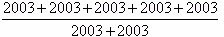Monday, March 2, 2009

Week 4 - Problem 4Figures I, II, III and IV are squares. The circumference of square I is 16m and the circumference of square II is 24m. Find the circumference of square IV.

A. 56m B. 60m C. 64m D. 72m E. 80m

Week 4 - Problem 3

The area of a rectangle equals 1. What is the area of the triangle, which is cut off from the rectangle by the line connecting the midpoints of the two adjacent sides?

A. 1/3 B. 1/4 C. 2/5 D. 3/8 E. 1/8

Saturday, February 28, 2009

Week 4 - Problem 2

The combination for opening a safe is a three – digit number made up of different digits. How many different combinations can you make using only digits 1, 3, and 5?

A) 2 B) 3 C) 4 D) 5 E) 6

Week 4 - Problem 1

The numbers 34 and 142 have the same sum of their digits (3+4=7 and 1+4+2=7). What is the first number greater than 2007 such that the sum of its digits is the same as the sum of the digits of 2007?

A) 2016 B) 2115 C) 2008 D) 7002 E) 2070

Monday, February 23, 2009

Week 3 - Problem 6

The fractionequals to

a) 2003    b) 1/3   c) 3    d) 5/2    e) None

Week 3 - Problem 5

Betty likes calculating the sum of the digits that she sees on her digital clock (for instance, if the clock shows 21:17, then Betty gets 11). What is the biggest sum she can get if the clock is a 24-hour clock?

A) 24 B) 36 C) 19 D) 25 E) another answer

Saturday, February 21, 2009

Week 3 - Problem 4

Rachel opened her math book and found that the sum of the facing pages was 243. What pages did she open to?

Week 3 - Problem 3

A math student interviewed 50 fifth graders. 41 students said they liked peanut butter sandwiches, 35 liked jam sandwiches, and 30 liked both on their sandwiches. How many students like neither?

Thursday, February 19, 2009

Week 3 - Problem 2

Sophie draws kangaroos: a blue one, then a green, then a red, then a black, a blue, a green, a red, a black, and so on…What color is the 29th kangaroo?

A) blue B) green C) red D) black E) it’s impossible to know

Week 3 - Problem 1

The sum of the numbers in each ring below should be 55. What is the value of A?

A) 9 B) 10 C) 13 D) 16 E) 17Wednesday, February 18, 2009

Challenge Problem

In the addition problem below, different letters represent different digits. What digit does A represent?

A A
+ A A
____
C A B

Challenge Problem

Mr. Chin went to a store where he spent one-half of his money and then \$14 more. He then went to another store where he spent one-third of his remaining money and then \$14 more. He then had no money left. How much did he have when he entered the first store?

Tuesday, February 17, 2009

Week 2 - Problem 6

Anna wrote a 2-digit number. Ben created a 4-digit number by copying Anna's number twice. Then Anna divided Ben's number by her number. What was the result she got?

A) 100 B) 101 C) 1000 D) 1001 E) 10

Week 2 - Problem 5

Bill thought of an integer number. Nick multiplied this number either by 5 or by 6. John added either 5 or 6 to Nick’s result. Last, Andrew subtracted either 5 or 6 from John’s result. The final result obtained was 73. What was Bill’s number?

A) 10 B) 11 C) 12 D) 14 E) 15

Monday, February 16, 2009

Week 2 - Problem 4

Harry Potter let an owl out at 7:30 a.m. to deliver an important message to his friend Ron. The owl delivered the envelope at 9:10 a.m. An owl flies 4 km in 10 minutes. What was the distance between Harry and Ron?

A) 14 km B) 20 km C) 40 km D) 56 km E) 64 km

Week 2 - Problem 3

What is the 2007th letter in the sequence KANGAROOKANGAROOKANG… ?

A) K

B) A

C) N

D) R

E) O

Week 2 - Problem 2

There were 60 birds on three trees. At some moment 6 birds flew away from the first tree, 8 birds flew away from the second tree, and 4 birds flew away from the third tree. After that, it turned out that the number of birds on each tree was the same. How many birds were there on the second tree in the beginning?

A) 26 B) 24 C) 22 D) 21 E) 20

Week 2 - Problem 1The square in the figure is a mini-sudoku: the numbers 1, 2, and 3 must be written in the cells so that each of them appears in each row and in each column. Harry started to fill in the square. In how many ways can he complete the task?

A) 1 B) 2 C) 3 D) 4 E) 5

CHALLENGE PROBLEM

ABCD represents a four-digit number. The product of its digits is 70. What is the largest four-digit number that ABCD can represent?
CHALLENGE PROBLEM

A prime number is a whole number, greater than 1, that is divisible only by itself and 1. Some examples of prime numbers are 2,3,5,7,11, and 13. What is the largest prime number, P, such that 9 times P is less than 400?